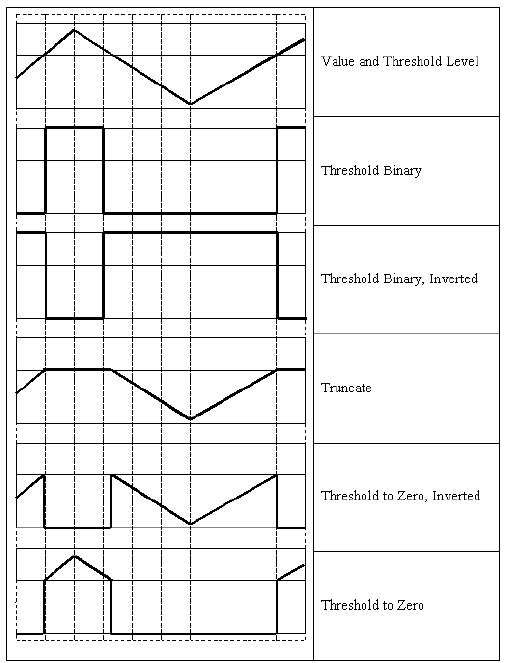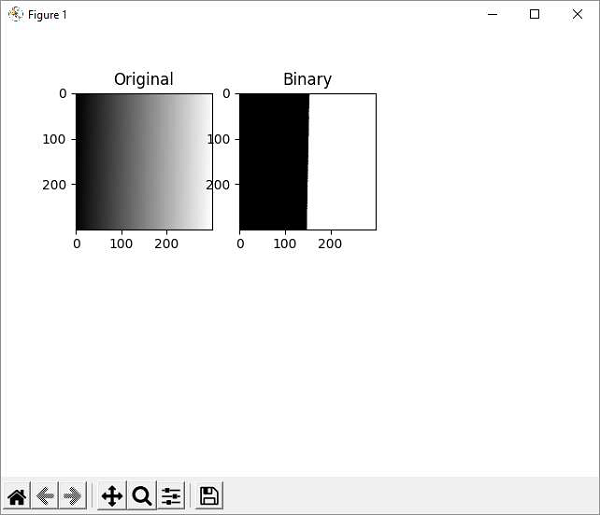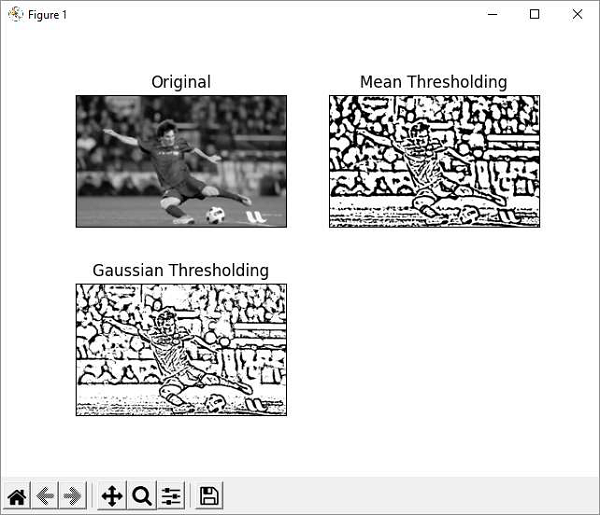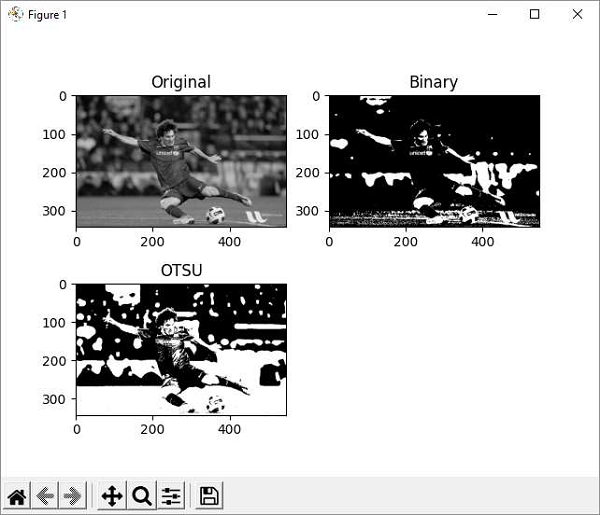# OpenCV Python - Image Threshold

In digital image processing, the thresholding is a process of creating a binary image based on a threshold value of pixel intensity. Thresholding process separates the foreground pixels from background pixels.

OpenCV provides functions to perform simple, adaptive and Otsu’s thresholding.

In simple thresholding, all pixels with value less than threshold are set to zero, rest to the maximum pixel value. This is the simplest form of thresholding.

The cv2.threshold() function has the following definition.

cv2.threshold((src, thresh, maxval, type, dst)


## Parameters

The parameters for the image thresholding are as follows −

• Src: Input array.
• Dst: Output array of same size.
• Thresh: Threshold value.
• Maxval: Maximum value.
• Type: Thresholding type.

## Types of Thresholding

Other types of thresholding are enumerated as below −

Sr.No Type & Function
1

cv.THRESH_BINARY

dst(x,y) = maxval if src(x,y)>thresh 0 otherwise

2

cv.THRESH_BINARY_INV

dst(x,y)=0 if src(x,y)>thresh maxval otherwise

3

cv.THRESH_TRUNC

dst(x,y)=threshold if src(x,y)>thresh src(x,y) otherwise

4

cv.THRESH_TOZERO

dst(x,y)=src(x,y) if src(x,y)>thresh 0 otherwise

5

cv.THRESH_TOZERO_INV

dst(x,y)=0 if src(x,y)>thresh src(x,y)otherwise

These threshold types result in operation on input image according to following diagram −The threshold() function returns threshold used and threshold image.

Following program produces a binary image from the original with a gradient of grey values from 255 to 0 by setting a threshold to 127.

## Example

Original and resultant threshold binary images are plotted side by side using Matplotlib library.

import cv2 as cv
import numpy as np
from matplotlib import pyplot as plt
ret,img1 = cv.threshold(img,127,255,cv.THRESH_BINARY)

plt.subplot(2,3,1),plt.imshow(img,'gray',vmin=0,vmax=255)
plt.title('Original')
plt.subplot(2,3,2),plt.imshow(img1,'gray',vmin=0,vmax=255)
plt.title('Binary')
plt.show()


## OutputThe adaptive thresholding determines the threshold for a pixel based on a small region around it. So, different thresholds for different regions of the same image are obtained. This gives better results for images with varying illumination.

The cv2.adaptiveThreshold() method takes following input arguments −

cv.adaptiveThreshold( src, maxValue, adaptiveMethod, thresholdType, blockSize, C[, dst] )


The adaptiveMethod has following enumerated values −

• cv.ADAPTIVE_THRESH_MEAN_C − The threshold value is the mean of the neighbourhood area minus the constant C.

• cv.ADAPTIVE_THRESH_GAUSSIAN_C − The threshold value is a Gaussianweighted sum of the neighbourhood values minus the constant C.

## Example

In the example below, the original image (messi.jpg is applied with mean and Gaussian adaptive thresholding.

import cv2 as cv
import numpy as np
from matplotlib import pyplot as plt
img = cv.medianBlur(img,5)
cv.THRESH_BINARY,11,2)
cv.THRESH_BINARY,11,2)
titles = ['Original', 'Mean Thresholding', 'Gaussian Thresholding']
images = [img, th1, th2]
for i in range(3):
plt.subplot(2,2,i+1),plt.imshow(images[i],'gray')
plt.title(titles[i])
plt.xticks([]),plt.yticks([])
plt.show()


## Output

Original as well as adaptive threshold binary images are plotted by using matplotlib as shown below −## Example

OTSU algorithm determines the threshold value automatically from the image histogram. We need to pass the cv.THRES_OTSU flag in addition to the THRESH-BINARY flag.

import cv2 as cv
import numpy as np
from matplotlib import pyplot as plt
# global thresholding
ret1,img1 = cv.threshold(img,127,255,cv.THRESH_BINARY)
# Otsu's thresholding
ret2,img2 = cv.threshold(img,0,255,cv.THRESH_BINARY+cv.THRESH_OTSU)
plt.subplot(2,2,1),plt.imshow(img,'gray',vmin=0,vmax=255)
plt.title('Original')
plt.subplot(2,2,2),plt.imshow(img1,'gray')

plt.title('Binary')
plt.subplot(2,2,3),plt.imshow(img2,'gray')
plt.title('OTSU')
plt.show()


## Output

The matplotlib’s plot result is as follows −